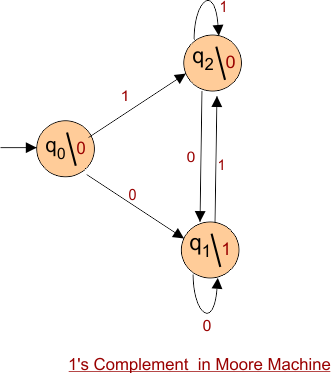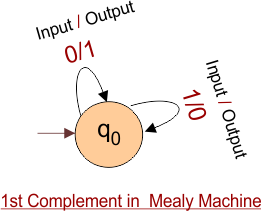Select Page

# Differences in Moore and Mealy Machine

Both Moore and Mealy Machines accept the regular languages and provide the output. There are some basic differences in Moore and Mealy Machines. Let explain,

 Sr. Moore Machine Mealy Machine 1. Output in Moore Machine is placed with in each state. Output in Mealy Machine is placed each transition. 2. Output of Moore Machine depends only upon present state.Output depends on present state as well as present input.3. λ is the output function which maps Q → O. Where Q is state and O is output. ‘λ’ is the output function which maps Q×∑→ O. Where Q is state and O is output. 4. If input changes, output does not change because output just depend on State. If input changes, output also changes. Because output depend on State and input. 5. Diagram of Moore Machine which gives first complement is given under, Diagram of Mealy Machine which gives first complement is given under, 6. After conversion from Moore to Mealy machine, numbers of state are always decreased. After conversion from Mealy  to Moore machine, number of state can increased. 7. Output is synchronized with clock. Moore Machines react slower to inputs (1 clock cycle later). Output is not synchronized with clock. Mealy Machines react faster to inputs. 8. It is Easy to design. It is difficult to design. 9. If the length of input string is “N” then the length of output string is “N+1” If the length of input string is “N” then the length of output string is also “N”
Help Other’s By Sharing…